# Orthogonal

## Plus, Minus: A Gentle Introduction to the Physics of Orthogonal

 B B A A A A A

For the past year or so I’ve been spending most of my waking hours in a place where light, matter, energy and time obey different laws of physics than those that rule our own universe. Studying the way things move and interact under these alternative laws reveals some familiar behaviour, some strange and eerily beautiful phenomena, and some terrifying risks.

To reach what I will call the Riemannian universe involves nothing more than changing a minus sign to a plus sign in a simple equation that governs the geometry of space-time. And curiously enough, although the consequences sometimes seem bizarre, the basic laws here can be understood more easily and intuitively than those that apply in the real world.

We have known for more than a hundred years that the best way to understand time in our universe is to think of it as combining with the familiar three dimensions of space to form a four-dimensional space-time, which obeys its own distinctive geometrical laws. While Newton saw time as an absolute, universal quantity that marched forward in lock-step at an identical rate for everyone, since Einstein we’ve realised that the passage of time measures an aspect of our paths through four-dimensional space-time that is very similar to the length of a path in space.

Nobody is surprised that different paths through space between the same two points can have different lengths. If I drive by the most direct route from Perth to Sydney, while you take a detour to Darwin along the way, it’s hardly shocking to suggest that our odometers will show us having travelled different distances. We now understand that detours in space-time can have an analogous effect on the passage of time. If you “travel” from New Year’s Eve in 2050 to New Year’s Eve in 2060 by staying on the Earth, whereas I, though joining you on both occasions, spend the intervening period travelling to Alpha Centauri and back, I will have aged about six years, compared to your ten.

While detours in space lead to longer paths, detours in space-time lead to shorter ones. The reason for this can be expressed quite simply: when intervals of time are being measured, the space-time version of Pythagoras’s Theorem involves a difference rather than a sum, a minus sign rather than a plus sign.

Pythagoras’s Theorem states that the square of the hypotenuse of a right-angled triangle equals the sum of the squares of the other two sides. For example:The space-time version, however, replaces the sum of the squares with a difference:To keep the formula simple, we’ve made a choice of units in which time and space are treated on an equal footing: years for time, and light-years for space. Using two triangles like this, we can justify the earlier claim that a trip to Alpha Centauri, which is about four light-years away, could result in my ageing only six years compared to ten for you if you stayed at home:Of course these triangles don’t look correct to us: the hypotenuse that’s meant to be “3 years” long is represented by a line that is clearly longer than the vertical line that represents “5 years”. But that’s the whole point: the “Lorentzian” geometry of space-time is not the same as the geometry of space, so it’s impossible to portray every detail correctly on a sheet of paper.

It’s easy now to state the difference between our own universe and the alternative I’ve been exploring. In the Riemannian universe, the usual version of Pythagoras’s Theorem always applies, even when intervals of time are involved. Space-time diagrams for this universe can be drawn on a sheet of paper with perfect fidelity! And the naive intuition that tells you that a triangle with “5 years” on one side and “4 light-years” on another should look like this: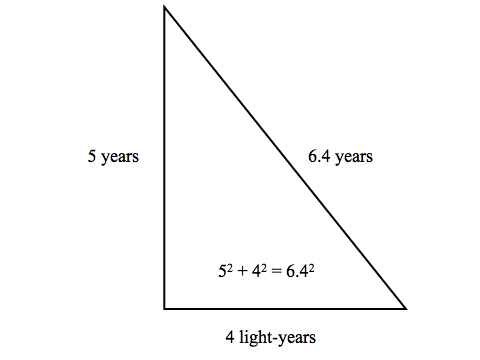is now perfectly correct. In the Riemannian universe, a detour to a distant star will always mean more time passing for the travellers, not less.

Interstellar voyages taking longer for the travellers than for everyone else might just sound annoying, but there are a multitude of other differences that flow from the same simple change, affecting everything from the appearance of the night sky to the stability of matter.

In our universe, the minus sign in the space-time version of Pythagoras’s Theorem means that as soon as the distance (measured in light-years) exceeds the time (measured in years), we end up with a negative number for the difference of squares. For example, if we think of travelling 5 light-years in 4 years, the formula implies that the elapsed time, squared, should be minus 9. Since no real number has a negative square, there is obviously something qualitatively different about such a hypothetical journey. And of course, what this turns out to be telling us is that nothing can actually cross such a large distance in such a short time.

In the Riemannian universe, there is no problem with the equivalent trip. The crew will measure the 5 light-year voyage as lasting 6.4 years, but to someone back home it will last just 4 years, and the ship will be seen as travelling at one-and-a-quarter times the speed of light. In the Riemannian universe, there is no universal speed limit.

In fact, in the Riemannian universe there is no universal speed of light either, so the terms “light-year” and “the speed of light” are really only appropriate in our own universe. In the Riemannian universe, what we need to use instead of “the speed of light” is a conversion factor from times to distances that makes the Pythagorean formula take on its nice simple form. Just as you would find that Pythagoras’s Theorem doesn’t work if you measure distances north-to-south in kilometres and distances east-to-west in miles, in the Riemannian universe it’s a matter of determining, experimentally, what units you need to use for times and distances to make the theorem apply.

What would it be like to live in a universe where light travels at different speeds? Of course it does this in our own universe when passing through transparent materials, such as glass, but in the Riemannian universe it will happen even in the vacuum of space. We perceive different wavelengths of light as different colours; in the Riemannian universe, those different wavelengths will always travel at different speeds.

We can find the relationship between wavelength and speed by noting that the separation of light’s wavefronts in space-time will always be the same, whatever the light’s speed. Why? We expect physics in our own universe not to favour any direction in three-dimensional space; if we take a laser and aim it south-east, we would expect it to produce light with exactly the same properties as if we’d aimed it north, or north-west. Similarly, the underlying physics in the Riemannian universe should be indifferent to the direction in space-time that characterises the light’s velocity.

Suppose the space-time diagram for a moderately fast pulse of light looks like this:Here the “path” we have drawn for the pulse of light shows its overall motion through space over time. And just as the crests of a wave in water lie at right angles to the direction in which the wave is travelling, the wavefronts that mark the peaks of this light wave’s oscillations lie at right angles to its path.

The wavelength of the light is the distance between those wavefronts in space. Of course the direction we choose to call “space” depends on our own state of motion, so what we measure for the wavelength will also depend on that. In contrast, the separation of the wavefronts, measured at right angles to them, is a property of the light itself, completely independent of who’s doing the measuring.

We can find the wavelength for a slower pulse of light simply by rotating the whole stack of wavefronts from the previous diagram, without changing the separation between them: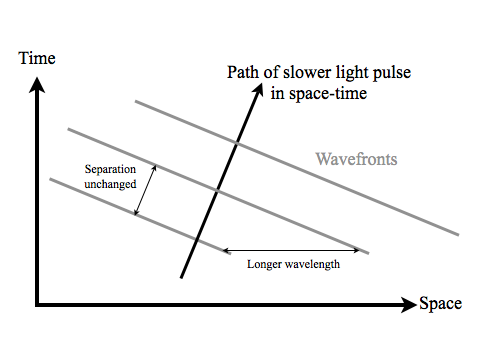So, light that is travelling more slowly will have a longer wavelength. If we translate this into our own language for the colours we perceive for different wavelengths, we can say that red light will be slower than violet light.

When you gaze up into the night sky in the Riemannian universe, the red light you see from each star will have taken years or even centuries longer to reach you than the violet light — so unless the star has no sideways motion at all, the different colours will be arriving from slightly different positions in the sky. Instead of appearing as single points of light, each star’s image will be spread out into a spectrum, or “colour trail”, with the angle between red and violet a direct measure of the star’s sideways velocity.

Apart from the absence of a universal speed limit, what can we say about the behaviour of matter in the Riemannian universe? To understand the most striking difference, we’ll first need to sketch some simple ideas from the physics of our own universe.

There are two useful quantities we associate with an object in motion: its momentum, and its kinetic energy. Probably the simplest way to characterise these is to imagine firing a bullet into some kind of barrier which has been designed to bring it to a halt by opposing its motion with a constant force. The bullet’s momentum will determine how long it takes to come to a stop, while its kinetic energy will determine how far it travels before doing so. Both of these quantities will depend only on the mass and speed of the bullet.

Since the advent of relativity, we’ve come to understand that, just as space and time are best seen as aspects of a single entity, space-time, the energy and momentum of a moving body are both aspects of a single geometrical object, known as the energy-momentum vector. That might sound horribly technical, but in fact it’s a very easy thing to picture. For a stationary object, the energy-momentum vector is just an arrow whose length is equal to the mass of the object, pointing in the time direction of space-time.If the same object is set in motion, the original arrow is simply tilted to reflect the amount of space the object crosses in a given time: a slight tilt for a slow-moving object, a greater tilt for a faster one.

In the diagram above, it might look as if the arrow has not merely been tilted but also increased in size. But that’s just an illusion created by drawing space-time on a page; if we apply the space-time version of Pythagoras’s Theorem to calculate the length of the tilted arrow, we’ll find that it has exactly the same length as the vertical arrow.

What use are these energy-momentum vectors? If you measure the extent in the time direction of an object’s energy-momentum vector, that tells you the object’s energy. If you measure the extent in the space direction, that tells you the object’s momentum.The one slightly tricky aspect here is that the extent of the energy-momentum vector in the time direction gives us what’s known as the total energy of the object, which includes not just its kinetic energy but also the energy associated with the object’s mass. So while a stationary object has zero momentum, just as you’d expect, it doesn’t have zero total energy; rather, its total energy is due entirely to its mass (which, as you’ll recall, is what determines the length of these arrows). When the same object is put into motion, the amount by which its total energy increases, above and beyond its “rest-mass energy”, is its kinetic energy.

Why haven’t we invoked the famous formula for energy from rest mass, E=mc2? In the kind of units we’re using, the speed of light, c, will always be equal to one (for example, one light-year per year), so we have E=m with no need for c2.

What happens in the Riemannian universe? Again, we draw an arrow whose length is equal to the object’s mass, and we give it an appropriate tilt in accordance with the object’s velocity: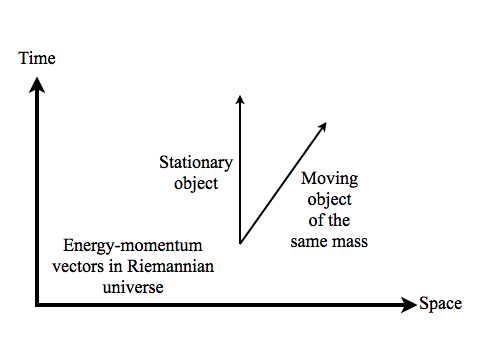This time, the tilt looks exactly like a tilt: we can see with our own eyes that the two arrows are the same length. The special version of Pythagoras’s Theorem for space-time has been replaced by the original version, so we can draw the Riemannian energy-momentum vectors on an ordinary sheet of paper and measure their length with an ordinary ruler.

How does this change affect the energy and momentum of a moving object?In the Riemannian universe, tilting the arrow causes it to span a smaller distance in the time direction than it did when the object was stationary. So the total energy of the object is less when it’s moving than when it’s at rest! We can still define the object’s kinetic energy as the difference between the two, but now it represents a decrease in energy, not an increase.

It sounds immensely strange to claim that a moving object will have less energy than a stationary one, but in fact the “upside-down” character of kinetic energy compared to total energy doesn’t show up at all in the simplest situations. All of the classical, Newtonian mechanics that we’re familiar with in our own universe continues to apply to slow-moving objects: you could play a game of pool in the Riemannian universe and have no idea that anything was different. The approximate formulas for momentum and kinetic energy for objects with low velocity are identical in the two universes — except for a minus sign for the kinetic energy, and nothing that happens on a pool table will reveal the significance of that. Kinetic energy is negative compared to rest-mass energy, but in our universe rest-mass energy only manifests itself in nuclear reactions. So maybe this odd quirk of the Riemannian universe would stay hidden in the everyday world, and only show up in arcane high-energy experiments?

But we’re forgetting something that’s very far from arcane: light! In our own universe, light has no rest mass, and the space-time version of Pythagoras’s Theorem allows the energy-momentum vector for light to have a corresponding length of zero. For this to be true, the total energy need not be zero, it only needs to be equal to the momentum (in units where the speed of light is one). That way, the extent of the vector in the space direction and the time direction cancel out in the difference of squares.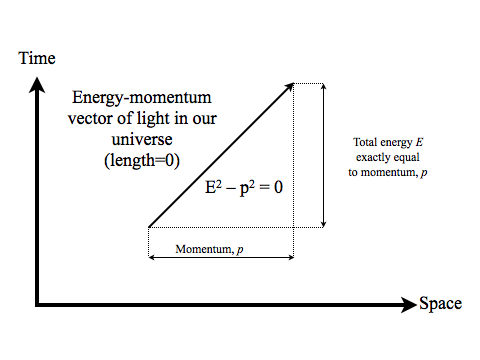In the Riemannian universe, this is impossible; the only way a vector can have a length of zero is if it spans a distance of zero in all directions. Having zero rest mass would mean having no energy or momentum at all. So in the Riemannian universe, light has rest mass, just like everything else.

Now, any process that creates light will need to obtain energy to do so from some other form, such as kinetic energy or chemical energy. But when kinetic energy is negative compared to total energy, “obtain” isn’t quite the right word. We need to obey the law of conservation of energy when light is created, but since the total energy of the light will be positive, the total energy of whatever created the light will need to decrease ... which means its kinetic energy will need to increase.

In other words, a system that creates light will undergo an increase in kinetic energy: its components will end up moving faster than before!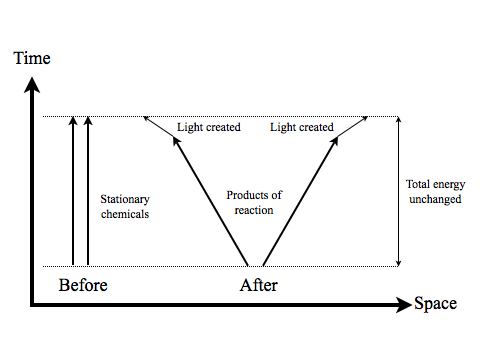To give a simple, concrete example, suppose we perform a chemical reaction that creates light. When we first mix the chemicals together, they’ll be more or less stationary (they’ll have some kinetic energy due to heat, but we’ll assume that’s negligible to start with). For the sake of simplicity, we’ll also assume that the two chemicals have equal mass. So, in the “before” diagram, their energy-momentum vectors are just two identical arrows pointing in the time direction.

The “after” diagram needs to include energy-momentum vectors for the light that is created, and for the products of the chemical reaction. We’ll assume that the products have the same mass as the reactants; that need not be true, but as we’ll see it’s certainly possible.

To fit all the vectors into the “after” diagram in such a way that the total energy is unchanged, the energy-momentum vectors for the products need to be tilted. In other words, the products will need to be moving: they need to have gained kinetic energy and lowered their total energy, in order to supply the total energy for the light that has been generated.

Kinetic energy created in a chemical reaction manifests as heat, so the reaction will produce both light and heat. But while light and heat are also produced in our universe when we burn any kind of fuel, in that case they’re created at the expense of a change in chemical energy, which shows up as a (very tiny) difference in the mass of the products compared to the reactants. Here, we’ve managed to create light and heat without relying on any such change! We chose to make the mass of the products exactly the same as that of the reactants, but the reaction could still have taken place if we’d made their mass either a bit higher or a bit lower.

In the Riemannian universe, the process of creating light can generate heat, with or without an accompanying change in chemical energy. And of course, chemical energy might sometimes be a more useful product than heat. Instead of absorbing light to power photosynthesis, plants in the Riemannian universe would emit light in order to store the resulting chemical energy.

The fact that the creation of light is accompanied by the creation of kinetic energy makes the Riemannian universe a perilous place. Life will need to master some delicate reactions in order to harvest energy from this process safely, and even the simplest building blocks of matter will face the threat of instability. In our universe, an atom or molecule can absorb a photon of light and enter a higher energy state in which it’s bound together less tightly; for example, ultraviolet radiation from sunlight can break some molecules apart. In the Riemannian universe, the same kind of process no longer requires an external source of radiation: matter in its most tightly bound state could emit its own light, and by doing so gain the kinetic energy to tear itself apart. Because of this effect, only certain structures will be stable.

What value is there in studying a universe ruled by laws different than our own? Of course we can contemplate the possibility that such a universe might actually exist — complete with inhabitants who ponder what life would be like if one of their plus signs was turned into a minus sign. But taking our own laws apart and seeing how they work under new assumptions also offers insights into physics in our own universe. We can deepen our understanding of many things — whether it’s a human society, a planet’s geology, the chemistry of life, or the most basic laws of physics — by striving to imagine how they might be different.

The Riemannian universe is brimming with danger and surprises. This essay has only scratched the surface, but I hope you’ve enjoyed this small taste of the delights it has to offer.Orthogonal / Plus, Minus: A Gentle Introduction to the Physics of Orthogonal / created Wednesday, 15 September 2010